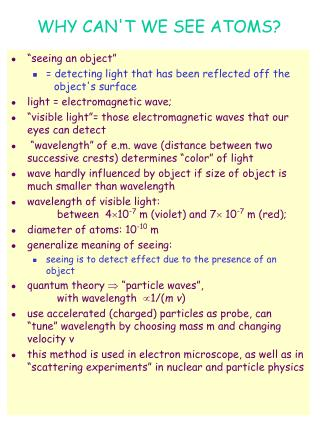DownloadDownload PresentationWHY CAN'T WE SEE ATOMS?

# WHY CAN'T WE SEE ATOMS?

Télécharger la présentation## WHY CAN'T WE SEE ATOMS?

- - - - - - - - - - - - - - - - - - - - - - - - - - - E N D - - - - - - - - - - - - - - - - - - - - - - - - - - -
##### Presentation Transcript

1. WHY CAN'T WE SEE ATOMS? • “seeing an object” • = detecting light that has been reflected off the object's surface • light = electromagnetic wave; • “visible light”= those electromagnetic waves that our eyes can detect • “wavelength” of e.m. wave (distance between two successive crests) determines “color” of light • wave hardly influenced by object if size of object is much smaller than wavelength • wavelength of visible light: between 410-7m (violet) and 7 10-7 m (red); • diameter of atoms: 10-10m • generalize meaning of seeing: • seeing is to detect effect due to the presence of an object • quantum theory  “particle waves”, with wavelength 1/(m v) • use accelerated (charged) particles as probe, can “tune” wavelength by choosing mass m and changing velocity v • this method is used in electron microscope, as well as in “scattering experiments” in nuclear and particle physics

2. WHAT IS INSIDE AN ATOM? • GREEK MODEL OF ATOM • atom = small, unchangeable particles; come in many varieties (corresponding to different “elements”) • move and combine in various ways. • this picture sufficient to describe all observations of early chemistry and physics (up to about middle of 19th century); • late 19th century: indications that there may be more: • observation of ions (``charged atoms''); • regularity seen in periodic table of elements due to atoms made up of smaller constituents? • where does electric charge come from? • THOMSON'S MODEL OF ATOM • (“RAISIN CAKE MODEL”): • J.J. Thomson (1897): discovery of electron; electron = charged particle, much less massive (2000 times) than lightest known ion; • appeared to come out of matter - part of atoms? • Thomson's atom model: • atom = sphere of positive charge (diameter 10-10 m), • with electrons embedded in it, evenly distributed (like raisins in cake)

3. RUTHERFORD MODEL OF ATOM • RUTHERFORD MODEL OF ATOM • (“PLANETARY MODEL”): • observations in “scattering experiments” (Geiger, Marsden, Rutherford, 1906 - 1911): measured angular distribution of scattered particles did not agree with expectations from Thomson model (only small angles expected), • but did agree with that expected from scattering on small, dense positively charged nucleus with diameter 10-14 m , surrounded by electrons at distance  10-10 m; • “planetary model”: • positive charge concentrated in nucleus (10-14 m); • negative electrons in orbit around nucleus at distance 10-10 m ; • electrons bound to nucleus by “electric” force; • problem with planetary atom model: electron orbiting in circular or elliptic orbit would lose energy by radiation orbit decays, and atoms would be unstable (lifetime  10-10 sec) • we would not exist to think about this!! • new theory needed to explain contradictions - Quantum Theory

4. CHEMICAL SYMBOLS ANDFORMULAE • chemical elements, and their atoms, are denoted by chemical symbols of one or two letters, (from their Latin or Greek name), e.g.: • O = oxygen (Oxygenium = acid maker) • Fe = iron (Ferrum) • K = potassium (Kalium) • Na = sodium (Natrium) • H = hydrogen (Hydrogenium = water maker) • chemical compounds, and their molecules, are denoted by their chemical formula chemical formula gives composition, e.g. • H2 O= water molecule, contains 2 hydrogen and one oxygen atoms • C O2 = carbon dioxide, contains one carbon and 2 oxygen atoms • O3 = ozone, contains 3 oxygen atoms • NaCl = sodium chloride = table salt • CnH2n+2= “alkane”, the simplest group of “hydrocarbon” compounds, such as: • C H4 = methane, • C2 H6 = ethane, • C3 H48 = propane, • C4 H10 = butane, • C8 H18 = octane, etc. • C2 H5 OH= ethanol, ethyl alcohol

5. chemical reactions • atoms can bond together to form compounds; • compounds can react with each other to make new compounds; • some chemical reactions: • oxidation = formation of new compound by bonding with oxygen, e.g. • burning of carbon: C + O2 CO2 + energy • burning of methane: CH4 + 2O2 CO2 + 6H2 O + energy • respiration: C2 H12 O6 + 6O2 6CO2 + 6H2O + energy respiration is the process by which animals retrieve energy stored in glucose, breathing in oxygen produced by plants, • Photosynthesis = mechanism by which plants convert energy of sunlight into energy stored in carbohydrates, generating oxygen as a “by-product” reaction: solar energy + CO2 + H2O  carbohydrates + O2